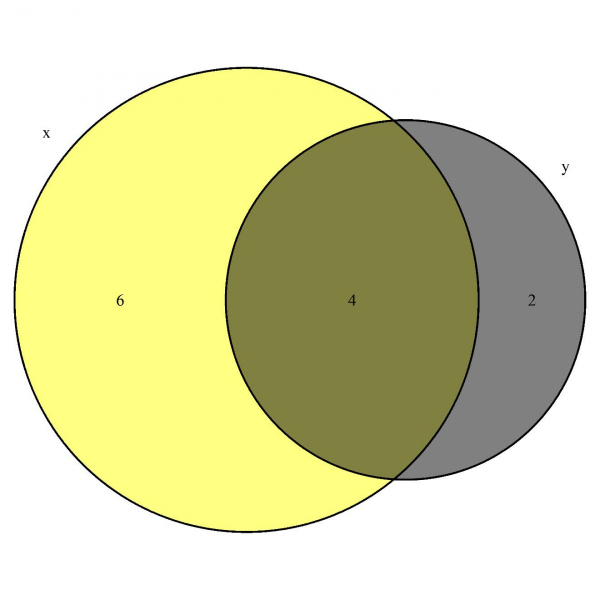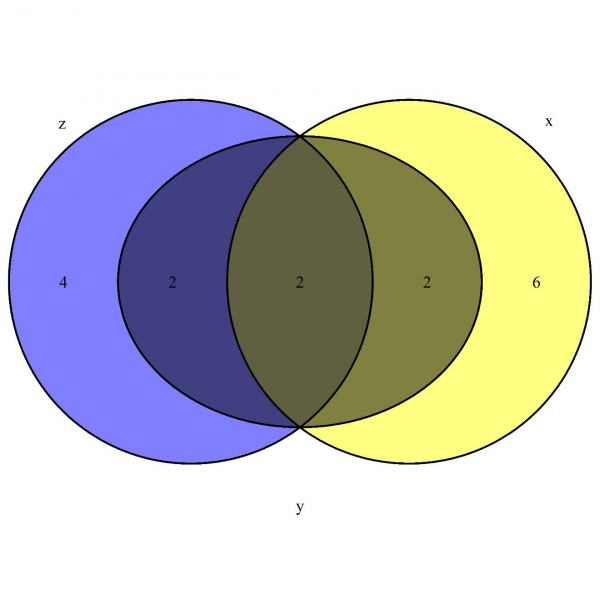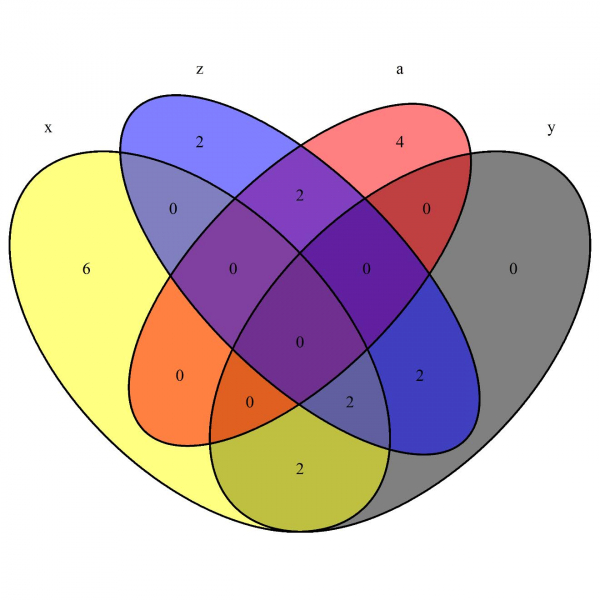# How to create colored Venn Diagrams?

Reading transparent Venn Diagrams is a little difficult due to transparency in all areas, therefore, we should create Venn Diagrams that has different color in each of the area. This will help us to easily read the diagram and also the diagram will be more appealing to viewers. We can do this by using venn.diagram function of VennDiagram package.

## Example

install.packages(“VennDiagram”)
library(VennDiagram)

Creating the colored Venn Diagram (Note: The colored diagram will be saved in default folder for R in your system, mostly it is documents folder) −

venn.diagram(list(x=1:10,y=7:12),fill=c("yellow","black"),
+ filename = "Example1.tiff")

## Outputvenn.diagram(list(x=1:10,y=7:12,z=9:16),fill=c("yellow","black","blue"),
+ filename = "Example2.tiff")

## Outputvenn.diagram(list(x=1:10,y=7:12,z=9:16,a=15:20),fill=c("yellow","black","blue","red"),
+ filename = "Example3.tiff")

## Output